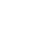Ordinary differential equations of the probability functions of the Benktander kind II distribution with b = 1 and its properties
The idea of convolution is the sum of independent and identically distributed (iid) random variables and the structure of linear combination of random variables. The cases of ordinary differential equation (ODE) of the convolution of probability distributions of mixture of Benktander distribution of the second kind have been studied. Moreover, the ODE of quantile function (QF), survival function (SF), hazard function (HF) and reversed hazard function (RHF) of convoluted probability distributions has been considered. We obtain explicit forms for the densities and distribution functions for Benktander distribution of the second kind, as well as their moments and related parame-ters. We derive basic properties of these laws and illustrate their modeling possible using a simulated data.
Publishing Year